#DAY 30
0

## [Day-30] Transfer learning & Model Serving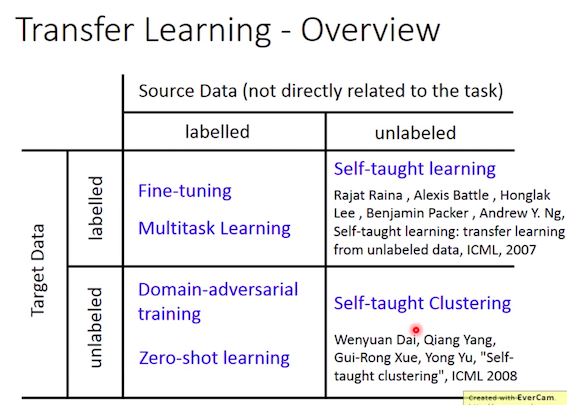source

``````with open('pre_trained.json','r') as f:

model = tf.keras.models.model_from_json(model_json)
model.summary()
``````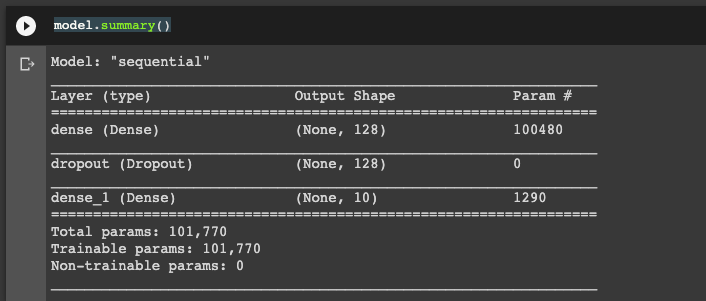``````app=Flask(__name__)
@app.route('/api/ml/<string:img>',methods=['POST'])

def classify_img(img):
classes = ['T-shirt/top','Trouser','Pullover','Dress','Coat','Sandal','Shirt','Sneaker','Bag','Ankle boot']
prediction = model.predict([image.reshape(-1,28*28)])

return jsonify({'object_detect':classes[np.argmax(prediction)]})

``````

``````r = requests.post("http://172.28.0.2:5000/api/ml/<image_name>")
print(r.text)
``````

``````(x_train,y_train),(x_test,y_test) = tf.keras.datasets.fashion_mnist.load_data()
for i in range(3):
imsave(name='/content/{}.png'.format(i),arr=x_test[i])
``````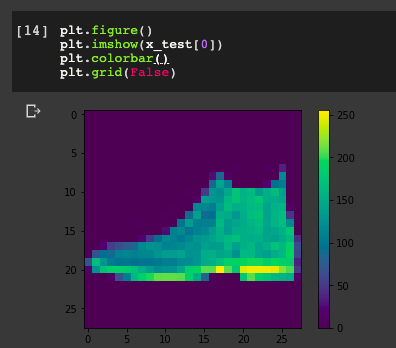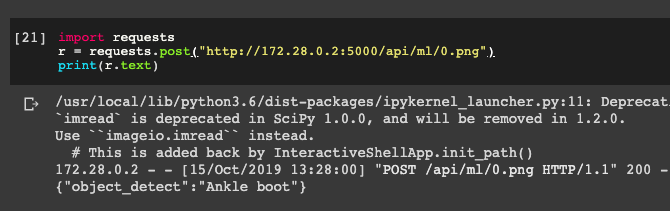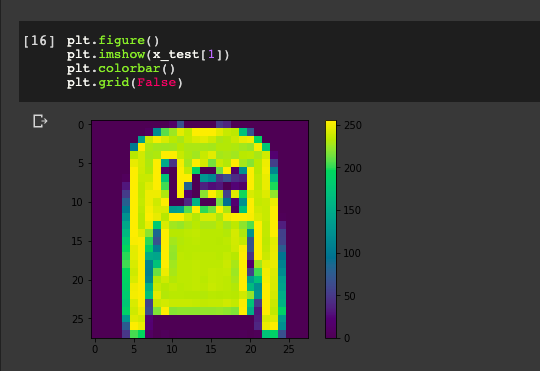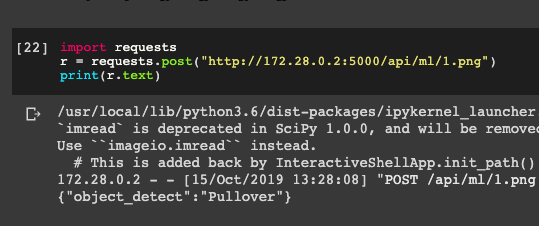#### 一日一梗圖：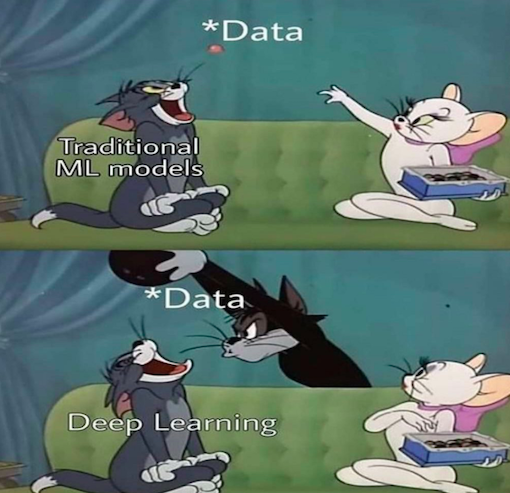source